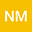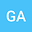A family of novel exact solutions to $(2+1)$-dimensional Boiti-Leon-Manna-Pempinelli equation
••In this manuscript, some novel exact traveling wave solutions are constructed for $(2+1)$-dimensional Boiti-Leon-Manna-Pempinelli(BLMP) equation. The analytical techniques, namely extended rational sine-cosine method and extended rational sinh-cosh method are utilized for constructing the new solitary wave solutions of BLMP equation. The proposed techniques provides different types of solutions which are expressed in terms of singular periodic wave, solitary waves, bright solitons, dark solitons, periodic wave and kink wave solutions with specific values of parameters.